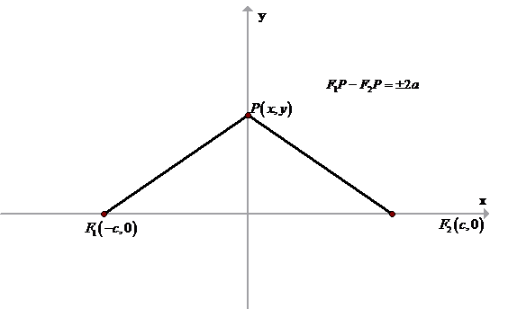# To use the definition of a hyperbola to derive the equation x 2 a 2 − y 2 b 2 = 1 , c 2 = a 2 + b 2 for a hyperbola with foci ( ± c , 0 )### Single Variable Calculus: Concepts...

4th Edition
James Stewart
Publisher: Cengage Learning
ISBN: 9781337687805### Single Variable Calculus: Concepts...

4th Edition
James Stewart
Publisher: Cengage Learning
ISBN: 9781337687805

#### Solutions

Chapter B, Problem 51E
To determine

## To calculate: To use the definition of a hyperbola to derive the equation x2a2−y2b2=1 , c2=a2+b2 for a hyperbola with foci (±c,0)

Expert Solution

Equation of hyperbola is derived

### Explanation of Solution

Given information: Equation of hyperbola is x2y2=1

Formula Used:

Hyperbola is the set of all the points in the plane whose difference from two points (the foci) is constant

Distance between two points is given as

D=(x1+x2)2+(y1+y2)2

Calculation:

Let us consider the below figureFrom above figure,

F1P=(x+c)2+y2F2P=(xc)2+y2

Also, given that thesum of the distances from point P(x,y) on the ellipse to the foci is 2a

Thus,

F1P+F2P=±2a(x+c)2+y2+(xc)2+y2=±2a(x+c)2+y2=±2a(xc)2+y2

Squaring both sides of equation,

((x+c)2+y2)2=(±2a(xc)2+y2)2(x+c)2+y2=(2a)2+(xc)2+y24a(xc)2+y2x2+c2+2xc+y2=4a2+x2+c22xc+y24a(xc)2+y24xc=4a24a(xc)2+y2xc=a2a(xc)2+y2xca2=a(xc)2+y2

Again, squaring both sides of equation

(xca2)2=(a(xc)2+y2)2c2x2+a42a2cx=a2((xc)2+y2)c2x2+a42a2cx=a2(x2+c22cx+y2)c2x2+a42a2cx=a2x2+a2c22a2cx+a2y2c2x2+a4=a2x2+a2c2+a2y2c2x2a2x2=a2c2a4+a2y2(c2a2)x2=(c2a2)a2+a2y2b2x2=b2a2+a2y2b2x2a2y2=b2a2x2a2y2b2=1

, where c2=a2+b2

Conclusion:

Hence, equation of hyperbola is derived

### Have a homework question?

Subscribe to bartleby learn! Ask subject matter experts 30 homework questions each month. Plus, you’ll have access to millions of step-by-step textbook answers!